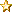###Author Topic: Data Type Gotcha!  (Read 75 times)

#### Nick_W

• Full Member
•• Posts: 215##### Data Type Gotcha!
« on: September 02, 2016, 04:51:03 pm »
I recently made an error that had me puzzled for a while. It didn't generate any compiler errors, or run time errors, it just gives wildly incorrect values.

What I did was assign an integer plus a float to an integer (stupid I know).

Here is the example:

Code: [Select]
`Dim Temp_Sample_Time As Integer = (2 * getTemperaturesamplingValue()) + 0.5`
Even if you do this:
Code: [Select]
`Dim Temp_Sample_Time As Integer = ((2 * getTemperaturesamplingValue()) + 0.5).ToInteger`or this:
Code: [Select]
`Dim Temp_Sample_Time As Integer = ((2 * getTemperaturesamplingValue().ToFloat) + 0.5).ToInteger`It doesn't work (but no errors).

Converting a Float to Integer using Float.ToInteger gives weird results (i'm sure it make sense from a bitwise point of view).

getTemperaturesamplingValue() returns an Integer in the range 0 to 16. The above code set "Temp_Sample_Time" to some huge number, and sent the program off into neverland.

Code: [Select]
`Dim Temp_Sample_Time As Float = (2 * getTemperaturesamplingValue()) + 0.5`
The above is correct (although I re-wrote this differently in the end).

Just a note to the wise.